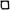Thomas C. Sideris

1.Nonexistence of global solutions to semilinear wave equations in high dimension. J. Diff. Eq. 52 3 (1984), 378-406.  Link.

2.Global behavior of solutions to nonlinear wave equations in three space dimensions. Comm. PDE. 8 12 (1983), 1291-1323.

3.Formation of singularities in solutions to nonlinear hyperbolic equations. Arch. Rat. Mech. Anal. 86 4 (1984), 369-381.

4.Formation of singularities in three-dimensional compressible fluids. Comm. Math. Phys. 101 (1985), 475-485.  Link.

5.(With M. Chipot) An upper bound for the waiting time for nonlinear degenerate parabolic equations. Trans. Am. Math. Soc. 288 1 (1985), 423-427.

6.Global existence of harmonic maps in Minkowski space. Comm. Pure Appl. Math. 42 1 (1989), 1-13.

7.Decay estimates for the three-dimensional inhomogeneous Klein-Gordon equation and applications. Comm. PDE. 14 14 (1989), 1421-1455.

8.(With M. Chipot) On the Abelian Higgs model. J. Appl. Math. Phys. 41 (1990), 105-109.

9.A decay estimate for the three-dimensional Klein-Gordon equation and global existence for nonlinear equations. Microlocal Analysis and Nonlinear Waves, M. Beals, R.B. Melrose, and J. Rauch, eds., IMA Volumes in Mathematics and Its Applications. 30 New York: Springer, 1991.

10.The lifespan of smooth solutions to the three-dimensional compressible Euler equations and the incompressible limit. Indiana Math. J. 40 2 (1991), 535-550.

11.The lifespan of 3D compressible flow. Centre de Mathematiques, Ecole Polytechnique. Expose No. V (1991).

12.(With R. Grauer) Numerical evidence for the finite-time blow up of 3-D incompressible ideal fluids with swirl. Phys. Rev. Let. 67 25 (1991), 3511-3514.

13.(With G. Ponce) Local regularity of nonlinear wave equations in three space dimensions. Comm. PDE. 18 1&2 (1993), 169-177.

14.(With G. Ponce, R. Racke, and E.S. Titi) Global stability of large solutions to the 3D Navier-Stokes equations. Comm. Math. Phys. 159 (1994), 329-341.

15.(With S. Klainerman) On almost global existence for nonrelativistic wave equations in 3D. Comm. Pure Appl. Math. 49 (1996), 307-322.  Link.

16.(With R. Grauer) Finite time singularities in ideal fluids with swirl. Physica D. 88 (1995), 116-232.

17.The null condition and global existence of nonlinear elastic waves. Inven. Math. 123 (1996), 323-342.  Link.

18.Long-time behavior of nonlinear elastic waves. Proc. Conf. on PDE, St. Jean de Monts (1995).

19.Delayed singularity formation in 2d compressible flow. Am. J. Math. 119 2 (1997), 371-422.

20.(With D. Iftimie and P. Gamblin) On the evolution of compactly supported planar vorticity. Comm. PDE. 42 9&10 (1999), 1709-1730.  Link.

21.Uniform decay estimates for some hyperbolic equations. Nonlinear wave equations (Providence, RI, 1998). Contemp. Math. 263. Amer. Math. Soc., Providence, RI, (2000), 185-195.

22.The null condition and global existence of nonlinear elastic waves. Differential equations and mathematical physics (Birmingham, AL, 1999).
AMS/IP studies in advanced mathematics, 16. Amer. Math. Soc., Providence, RI, (2000), 339-345.

23.Nonresonance and global existence of prestressed nonlinear elastic waves. Annals of Math. 151 (2000), 849-874. Link.

24.(With S.-Y. Tu) Global existence for systems of nonlinear wave equations in 3D with multiple speeds. SIAM J. Math. Anal. 33 (2001), 477-488.  Link.

25.(With X.-D. Liu) Convergence of the ghost fluid method for elliptic equations with interfaces. Math. Comp. 72 (2003), 1731-1746.  Link.

26.(With B. Thomases and D. Wang) Long time behavior of solutions to the 3D compressible Euler equations with damping. Comm. PDE. 28 7&8 (2003), 795-816. Link.

27.(With B. Thomases) Global existence for 3d incompressible and isotropic elastodynamics via the incompressible limit. Comm. Pure Appl. Math. 58 (2005), 750-788.  Link.

28.Nonlinear hyperbolic systems and elastodynamics. Phase Space Analysis of PDEs, Vol. II. Scuole Normale Superiore Pisa (2004), 451-485.  Link.

29.(With B. Thomases) Local energy decay for solutions of multi-dimensional isotropic symmetric hyperbolic systems. J. Hyperbolic Diff. Eq. 3 (2006), 673-690.  Link.

30.Dispersive estimates for solutions of multi-dimensional isotropic symmetric hyperbolic systems. Advanced Studies in Pure Mathematics, 47 1. Asymptotic Analysis and Singularities. Mathematical Society of Japan (2007), 55-63.
Link.

31.(With B. Thomases) Global existence for three-dimensional incompressible isotropic elastodynamics. Comm. Pure Appl. Math. 60 12 (2007), 1707-1730.  Link.

32.Energy splitting for solutions of multi-dimensional isotropic symmetric hyperbolic systems. Quart. Appl. Math.  68 1 (2010), 169-178.  Link.

33.(With L. Vega) Stability in L1 of circular vortex patches.  Proc. Amer. Math. Soc. 137 12 (2009),  4199-4202.  Link.

34.(With T. Grafke and R. Grauer) Turbulence properties and global regularity of a modified Navier-Stokes model. Physica D. 254 (2013), 18-23.   Link.

35.(With Z. Lei and Y. Zhou) Almost global existence for 2-D incompressible isotropic elastodynamics.  Trans. Am. Math. Soc. 367 11 (2015), 8175-8197.  Link.

36.Ordinary differential equations and dynamical systems.  Atlantis Press: Amsterdam-Paris (2013).   Link.

37.Spreading of the free boundary of an ideal fluid in a vacuum.  J. Diff. Eq. 257 (2014), 1-14.  Link.

38.(With B. Jonov)  Global and almost global existence of  small solutions to a dissipative wave equation in 3D with nearly null nonlinear terms.  Commun. Pure Appl. Anal. 14 4 (2015), 1407-1442.  Link.

39.Global existence and asymptotic behavior of affine motion of 3D ideal fluids surrounded by vacuum.  Arch. Rat. Mech. Anal.  225 1 (2017), 141-176.  Link.

40.(With F. Linares and G. Ponce)  Properties of solutions to the Camassa-Holm equation on the line in a class containing the peakons.  To appear in Adv. Studies in Pure Math. Link.

41.(With Jay Roberts and Steve Shkoller)  Affine motion of 2d incompressible fluids and flows in SL(2,R).  Link.

42.(With Steve Shkoller)  Global existence of near-affine solutions to the compressible Euler equations.  Arch. Rat. Mech. Anal.  234 1 (2017), 141-176. Link.

Research partially supported by the National Science Foundation